Open in App
Not now

# Class 8 RD Sharma Solutions – Chapter 27 Introduction to Graphs – Exercise 27.1

• Last Updated : 05 Mar, 2021

### Question 1. Plot the points (5, 0), (5, 1), (5, 8). Do they lie on a line? What is your observation?

Solution:

Initially take a point O on the graph and draw horizontal and vertical lines OX and OY.

In this graph x-axis and y axis 1 cm represents 1 unit.

First plot point (5, 0), we start from the origin O and move 5 cm along X – axis. The point is (5, 0).

Now plot point (5, 1), move 5 cm along X – axis and 1 cm along Y – axis. The point is (5, 1).

Now plot point (5, 8), move 5 cm along X – axis and 8 cm along Y – axis. The point is (5, 8).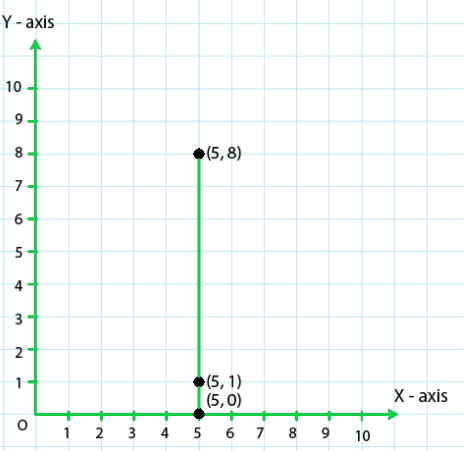From the above graph we observed that all points are having same X – coordinates, we can say that all points lie on a line parallel to the y-axis. Hence, all points lie on the same line.

### Question 2. Plot the points (2, 8), (7, 8) and (12, 8). Join these points in pairs. Do they lie on a line? What do you observe?

Solution:

Initially take a point O on the graph and draw horizontal and vertical lines OX and OY.

In this graph x-axis and y axis 1 cm represents 1 unit.

In order to plot point (2, 8), move 2 cm along X – axis from origin O and 8 cm along Y – axis. The point we arrive at is (2, 8).

To plot point (7, 8), move 7 cm along X – axis and 8 cm along Y – axis. The point we arrive at is (7, 8).

To plot point (12, 8), move 12 cm along X – axis and 8 cm along Y – axis. The point we arrive at is (12, 8).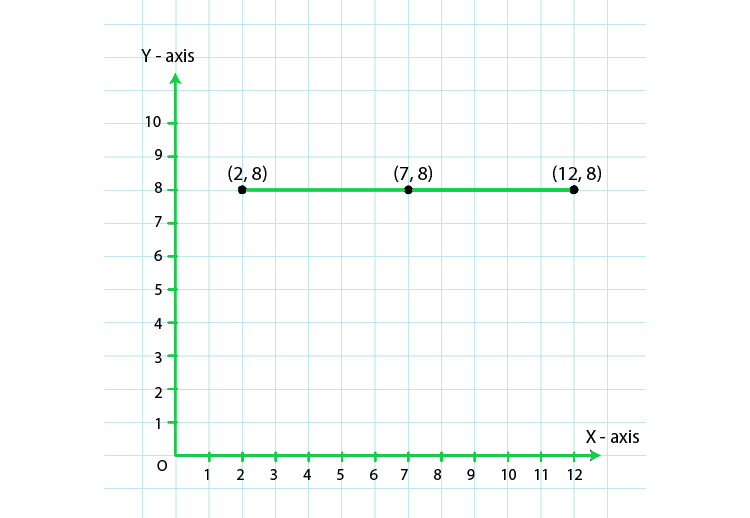From the above graph, we observed that all points are having same Y – coordinates, it can be seen that the points lie on a line parallel to the x-axis. Hence, all points lie on the same line.

### Question 3. Locate the points:

(i) (1, 1), (1, 2), (1, 3), (1, 4)

(ii) (2, 1), (2, 2), (2, 3), (2, 4)

(iii) (1, 3), (2, 3), (3, 3), (4, 3)

(iv) (1, 4), (2, 4), (3, 4), (4, 4)

Solution:

(i) (1, 1), (1, 2), (1, 3), (1, 4)

To plot these points,

Initially take a point O on the graph and draw horizontal and vertical lines OX and OY.

In this graph x-axis and y axis 1 cm represents 1 unit.

Initially to plot point (1, 1), move 1 cm along X – axis from origin O and 1 cm along Y – axis. The point we arrive at is (1, 1).

To plot point (1, 2), move 1 cm along X – axis and 2 cm along Y – axis. The point we arrive at is (1, 2).

To plot point (1, 3), move 1 cm along X – axis and 3 cm along Y – axis. The point we arrive at is (1, 3).

To plot point (1, 4), move 1 cm along X – axis and 4 cm along Y – axis. The point we arrive at is (1, 4)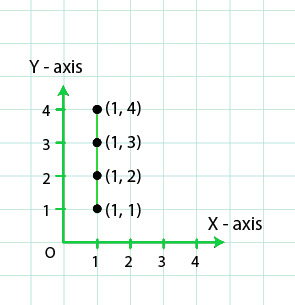(ii) (2, 1), (2, 2), (2, 3), (2, 4)

To plot these points,

Initially take a point O on the graph and draw horizontal and vertical lines OX and OY.

In this graph x-axis and y axis 1 cm represents 1 unit.

Initially plot point (2, 1), move 2 cm along X – axis and 1 cm along Y – axis. The point we arrive at is (2, 1).

To plot point (2, 2), move 2 cm along X – axis and 2 cm along Y – axis. The point we arrive at is (2, 2).

To plot point (2, 3), move 2 cm along X – axis and 3 cm along Y – axis. The point we arrive at is (2, 3).

To plot point (2, 4), move 2 cm along X – axis and 4 cm along Y – axis. The point we arrive at is (2, 4).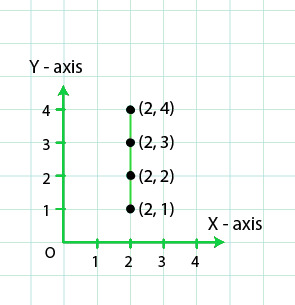(iii) (1, 3), (2, 3), (3, 3), (4, 3)

To plot these points,

Initially take a point O on the graph and draw horizontal and vertical lines OX and OY.

In this graph x-axis and y axis 1 cm represents 1 unit.

Initially plot point (1, 3), move 1 cm along X – axis and 3 cm along Y – axis. The point we arrive at is (1, 3).

To plot point (2, 3), move 2 cm along X – axis and 3 cm along Y – axis. The point we arrive at is (2, 3).

To plot point (3, 3), move 3 cm along X – axis and 3 cm along Y – axis. The point we arrive at is (3, 3).

To plot point (4, 3), move 4 0cm along X – axis and 3 cm along Y – axis. The point we arrive at is (4, 3).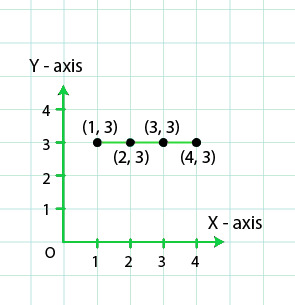(iv) (1, 4), (2, 4), (3, 4), (4, 4)

To plot these points,

Initially take a point O on the graph and draw horizontal and vertical lines OX and OY.

In this graph x-axis and y axis 1 cm represents 1 unit.

In order to plot point (1, 4), move 1 cm along X – axis and 4 cm along Y – axis. The point we arrive at is (1, 4).

To plot point (2, 4), move 2 cm along X – axis and 4 cm along Y – axis. The point we arrive at is (2, 4).

To plot point (3, 4), move 3 cm along X – axis and 4 cm along Y- axis. The point we arrive at is (3, 4).

To plot point (4, 4), move 4 cm along X – axis and 4 cm along Y – axis. The point we arrive at is (4, 4).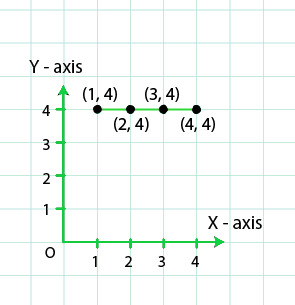### Question 4. Find the coordinates of points A, B, C, D.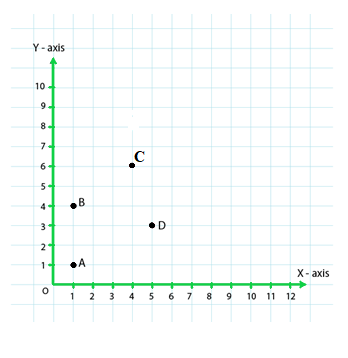Solution: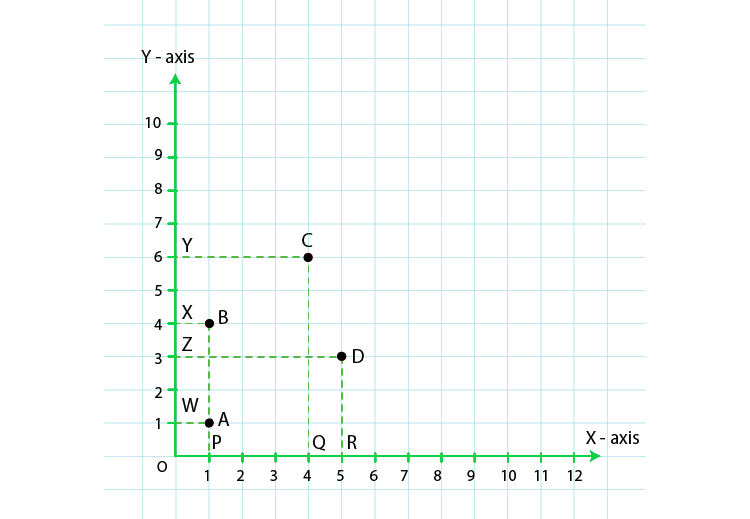Initially draw perpendiculars AP, BP, CQ and DR from A, B, C and D on the x-axis. Also, draw perpendiculars AW, BX, CY and DZ on the y-axis.

From the above figure we have observed that,

AW = 1 unit and AP= 1 unit

Therefore the coordinates of vertex A are (1, 1).

Similarly, BX=1 unit and BP= 4 units

Hence, the coordinates of vertex B are (1, 4).

CY = 4 units and CQ= 6 units

Therefore, the coordinates of vertex C are (4, 6).

DZ = 5 units and DR= 3 units

Hence, the coordinates of vertex D are (5, 3).

### Question 5. Find the coordinates of points P, Q, R and S.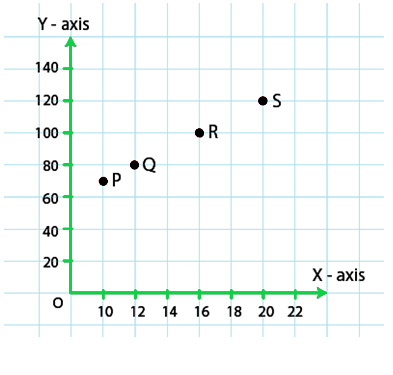Solution: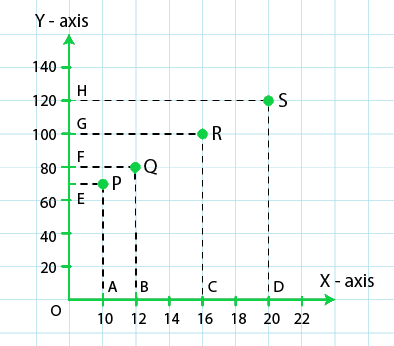Initially draw perpendiculars PA, QB, RC and SD from vertices P, Q, R and S on the X – axis and draw perpendiculars PE, QF, RG, and SH on the Y – axis from these points.

PE = 10 units and PA = 70 units

therefore, the coordinates of vertex P are (10, 70).

QF = 12 units and QB = 80 units

therefore, the coordinates of vertex Q are (12, 80).

RG = 16 units and RC = 100 units

therefore, the coordinates of vertex R are (16, 100).

SH = 20 units and SD = 120 units

therefore, the coordinates of vertex S are (20, 120).

### Question 6. Write the coordinates of each of the vertices of each polygon.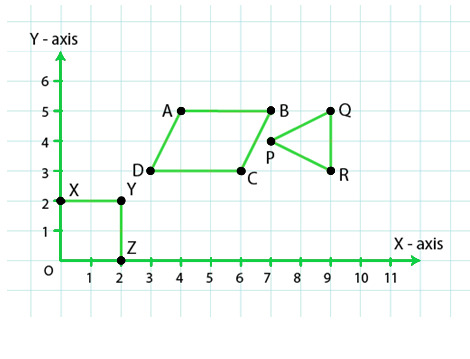Solution: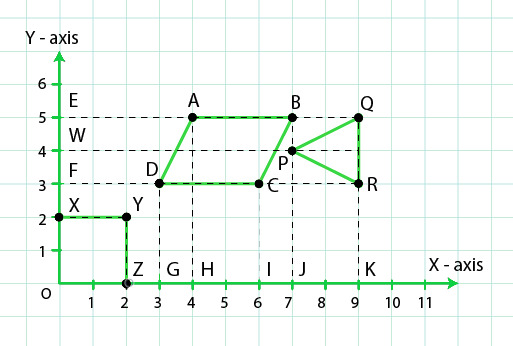From the figure, we have observed that,

O lies on the origin and the coordinates of the origin are (0, 0). So, the coordinates of O are (0, 0).

X lies on the Y – axis. So, the X – coordinate is 0. Hence, the coordinate of X is (0, 2).

YX is equal to 2 units and YZ is equal to 2 units. So, the coordinates of vertex Y are (2, 2).

Z lies on the X – axis. So, the Y – coordinate is 0. Hence, the coordinates of Z are (2, 0).

In polygon ABCD,

Draw perpendiculars DG, AH, CI and BJ from A, B, C and D on the X – axis.

Also, draw perpendiculars DF, AE, CF and BE from A, B, C and D on the Y – axis.

Now, from the figure we observed that,

DF = 3 units and DG = 3 units

Therefore, the coordinates of D are (3, 3).

AE = 4 units and AH = 5 units

Therefore, the coordinates of A are (4, 5).

CF = 6 units and CI = 3 units

Therefore, the coordinates of C are (6, 3).

BE = 7 units and BJ = 5 units

Therefore, the coordinates of B are (7, 5).

In polygon PQR,

Draw perpendiculars PJ, QK and RK from P, Q and R on the X – axis and draw perpendiculars PW, QE and RF from P, Q and R on the Y – axis.

Now, from the figure observed that,

PW = 7 units and PJ = 4 units

Therefore, the coordinates of P are (7, 4).

QE = 9 units and QK = 5 units

Therefore, the coordinates of Q are (9, 5).

RF = 9 units and RK = 3 units

Therefore, the coordinates of R are (9, 3)

### Question 7. Decide which of the following statements is true and which is false. Give reasons for your answer.

(i) A point whose x-coordinate is zero, will lie on the y-axis.

(ii) A point whose y-coordinate is zero, will lie on x-axis.

(iii) The coordinates of the origin are (0, 0).

(iv) Points whose x and y coordinates are equal, lie on a line passing through the origin.

Solution:

(i) A point whose x-coordinate is zero, will lie on the y-axis.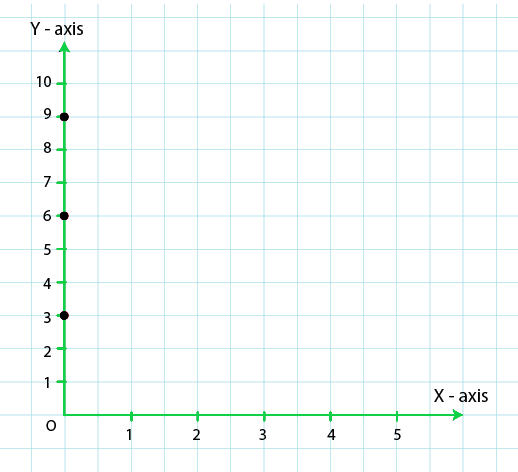From the figure we observed that,

For x = 0, we have x- coordinates as zero.

For example (0, 3), (0, 6), (0, 9)

These points will lie on y axis. Therefore we say that our given statement is true.

(ii) A point whose y-coordinate is zero, will lie on x-axis.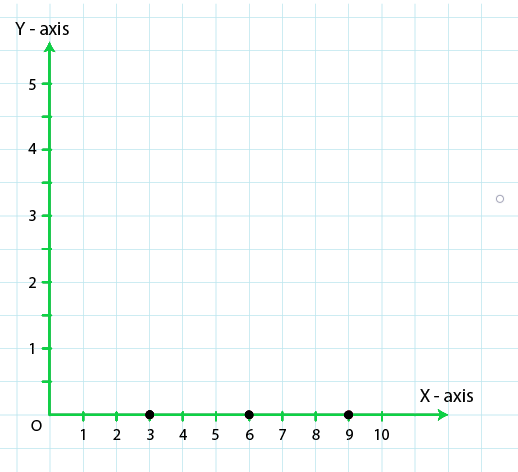A point whose y-coordinate is zero, will lie on x-axis.

For y = 0, we have y- coordinates as zero.

For example (3, 0), (6, 0), (9, 0)

These points will lie on x axis. Therefore we say that our given statement is true.

(iii) The coordinates of the origin are (0, 0).

Origin is intersection of x-axis and y-axis. This means that coordinates of the origin will be intersection of lines y = 0 and x = 0.

Hence, coordinates of origin are (0, 0).

Therefore given statement is true.

(iv) Points whose x and y coordinates (0, 0), (1, 1), (2, 2) etc are equal, lie on a line passing through the origin.

For above statement we can conclude that our statement satisfies the equation x = y.

For x = 0 and y = 0, this equation gets satisfied.

Therefore given statement is true.

My Personal Notes arrow_drop_up
Related Articles